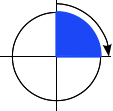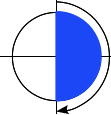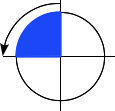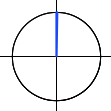/CSS

# <angle>

The `<angle>` CSS data type represents an angle value expressed in degrees, gradians, radians, or turns. It is used, for example, in `<gradient>`s and in some `transform` functions.

## Syntax

The `<angle>` data type consists of a `<number>` followed by one of the units listed below. As with all dimensions, there is no space between the unit literal and the number. The angle unit is optional after the number `0`.

Optionally, it may be preceded by a single `+` or `-` sign. Positive numbers represent clockwise angles, while negative numbers represent counterclockwise angles. For static properties of a given unit, any angle can be represented by various equivilent values. For example, `90deg` equals `-270deg`, and `1turn` equals `4turn`. For dynamic properties, like when applying an `animation` or `transition`, the effect will nevertheless be different.

### Units

`deg`
Represents an angle in degrees. One full circle is `360deg`. Examples: `0deg`, `90deg`, `14.23deg`.
`grad`
Represents an angle in gradians. One full circle is `400grad`. Examples: `0grad`, `100grad`, `38.8grad`.
`rad`
Represents an angle in radians. One full circle is 2π radians which approximates to `6.2832rad`. `1rad` is 180/π degrees. Examples: `0rad`, `1.0708rad`, `6.2832rad`.
`turn`
Represents an angle in a number of turns. One full circle is `1turn`. Examples: `0turn`, `0.25turn`, `1.2turn`.

## ExamplesA right angle: `90deg = 100grad = 0.25turn ≈ 1.5708rad`A flat angle: `180deg = 200grad = 0.5turn ≈ 3.1416rad`A right angle (counterclockwise): `-90deg = -100grad = -0.25turn ≈ -1.5708rad`A null angle: `0 = 0deg = 0grad = 0turn = 0rad`

## Specifications

Specification Status Comment
CSS Values and Units Module Level 3
The definition of '<angle>' in that specification.
Candidate Recommendation Initial definition.

## Browser compatibilityUpdate compatibility data on GitHub

Desktop
Chrome Edge Firefox Internet Explorer Opera Safari
Basic support 2 12 3.6 9 Yes 4
`deg` 2 12 3.6 9 Yes 4
`grad` 2 12 3.6 9 Yes 4
`rad` 2 12 3.6 9 Yes 4
`turn` Yes 12 13 9 Yes 10
Mobile
Android webview Chrome for Android Edge Mobile Firefox for Android Opera for Android iOS Safari Samsung Internet
Basic support Yes Yes Yes 4 Yes Yes Yes
`deg` Yes Yes Yes 4 Yes Yes Yes
`grad` Yes Yes Yes 4 Yes Yes Yes
`rad` Yes Yes Yes 4 Yes Yes Yes
`turn` Yes Yes Yes 14 Yes 10 Yes

© 2005–2018 Mozilla Developer Network and individual contributors.